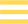# Orbital Period

### The Sidereal Period

The period of time taken for a body in orbit around another to complete a single orbital cycle around its parent body. In the case of planets, this is more colloquially known as a planet's 'year', but the concept of an orbital period can equally apply to moons orbiting planets, stars orbiting other stars, or any other set of objects in a similar relationship. This period is commonly measured against the background stars, a method that is technically described as a sidereal period.

The orbital period is a function of the orbiting body's distance from the focus of its orbit, with more distant objects having longer orbital periods. This relationship is formally described by Kepler's Third Law (which states that the square of the orbital period is proportional to the cube of the body's distance* from the object it is orbiting).

For example, Mercury orbits the Sun at a distance less than a third that of Earth and its orbital period is just 88 days, while Earth's own orbital period is just over 365 days (thus defining the year). Out in the depths of the Solar System, the planet Neptune, more than thirty times Earth's distance from the Sun, has a correspondingly extreme orbital period of some 60,182 days (or nearly 165 years).

### Other Orbital Factors and Periods

Technically speaking, no object in space actually orbits another, but rather both orbit their common centre of gravity, or barycentre. In familiar situations where one object is much more massive than the other, for example planets orbiting the Sun, or moons orbiting planets, this distinction is usually insignificant. Things can be different, though, in situations where both bodies are of comparable mass, as for example in binary systems containing two stars of similar mass. In cases like these, the components will pursue a mutual orbit around a point in space that lies between them. Nonetheless, it is still meaningful to calculate an orbital period in these situations, or at least a sidereal period measured against background stars.

Though an object's orbital period is usually synonymous with its sidereal period, there are other ways to calculate the period of an orbit. A synodic period, for example, is calculated relative to a third body, rather than the background stars. This may seem an abstract idea, but there is a familiar example: the phases of the Moon.

The Moon's phases depend on its position relative to both the Earth and Sun, so the orbital period between (for example) one Full Moon and the next is affected not only by the Moon's orbital motion around the Earth, but also the Earth's around the Sun. The practical result of this is that the Moon's sidereal orbital period (its time to complete a single orbit of Earth) is 27.3 days, while its synodic orbital period (the time taken to repeat the same phase, as seen from Earth) is slightly longer at 29.5 days.

* Since orbits are typically elliptical, this distance necessarily varies over time. 'Distance' here refers properly to the semi-major axis, the distance to a fixed, mathematically defined point on an orbital ellipse.